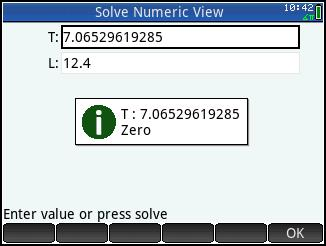Equation Library

## Description

The program eqnlib offers 47 equations for HP Prime’s Solver App. Just run the eqlib program, select the category that you are interested in, and select the equation within that category and you are off and running!

The equation selected will be loaded into the system variable E1. The program will show you what each variable represents in the solver. Keep this screen up as long as you like. When done, press [Enter], and you will be taken to the Numeric View of the Solve App. To see the equation, press the soft (Defn) key.

Categories include:
* Geometry (areas, volumes, surface areas)
* Finance (monthly loans, sales tax, break-even)
* Temperature Conversion (Fahrenheit to and from Celsius)
* Physics: Problems of Motion (linear distance, circular motion, pendulums, terminal & escape velocity)
* Sound (speed of sound, Doppler effect, loudness)
* Optics (Lens and Snell equations, spherical refraction)
* Electronics (2 resistors series and parallel, thermal noise, Ohm's Law)
* Astronomy (parallax, luminosity, Kepler's 3rd Law, time dilation)
* Great Circle Calculations
* Angle of Incidence Calculations
* Gases (Ideal and Boyle's Gas Laws, heat capacity, air density, isothermal expansion)
* Fluids (depth pressure, fluid flow, Bernoulli's Equation)

## Screenshots## Archive Contents

Name Size
eqnlib.hpprgm 20.3 KB
Equation Library for the HP Prime.pdf 249.4 KB
File Size
227.0 KB

Author
EdPi314
8 years, 4 months ago

Rating
No ratings.